• 试采用双线性插值对一幅图像进行放大，kx=2.3，ky=1.6，图像如下，根据原理设计，不要采用MATLAB封装好的函数。
• 图像双线性插值放大 f(x,y)=f(0,0)(1-x)(1-y)+f(1,0)x(1-y)+f(0,1)(1-x)y+f(1,1)xy
 图像双线性插值放大 f(x,y)=f(0,0)(1-x)(1-y)+f(1,0)x(1-y)+f(0,1)(1-x)y+f(1,1)xy
import  java.awt.Color;
import  java.awt.image.BufferedImage;
import  java.io.File;
import  java.io.IOException;

import  javax.imageio.ImageIO;

/**
*  图像双线性插值放大
*  f(x,y)=f(0,0)(1-x)(1-y)+f(1,0)x(1-y)+f(0,1)(1-x)y+f(1,1)xy
*  */
public  class  ch5_2  {

public  static  void  main(String[]  args)  throws  Exception  {
long  t1=System.currentTimeMillis();                //获取程序运行前的时间，单位毫秒
BufferedImage  nbi=zoomSxx(bi,4);                   //处理图像
ImageIO.write(nbi,  "jpg",  new  File("1b.jpg"));   //输出图像
long  t2=System.currentTimeMillis();                //获取程序运行后的时间
System.out.println("程序运行"+(t2-t1)+"毫秒");       //程序结束后进行提示

}

/**
*  图像双线性插值放大
*  @param  BufferedImage  bi     原始图像
*  @param  double  k             放大系数
*  @return  BufferedImage        变换后图像
*  */
public  static  BufferedImage  zoomSxx(BufferedImage  bi,  double  k)  {
int  w=(int)  (k*bi.getWidth());             //得到图像的宽度
int  h=(int)  (k*bi.getHeight());            //得到图像的高度
BufferedImage  nbi=new  BufferedImage(w,  h,  BufferedImage.TYPE_3BYTE_BGR);
//创建新图像（临时图像变量）宽度和高度跟原图相同
//循环遍历每一个像素点
for(int  y1=0;y1<h;y1++)  {
for(int  x1=0;x1<w;x1++)  {
//计算输出图像坐标(x1,y1)所对应原图中的插值像素值
int  rgb=zoomSxx(bi,k,x1,y1);
nbi.setRGB(x1,  y1,  rgb);        //设置输出图像坐标为（x,y）的像素值
}
}
return  nbi;
}

private  static  int  zoomSxx(BufferedImage  bi,  double  k,  int  x1,  int  y1)  {
double  xx=x1/k;
double  yy=y1/k;

int  x0=(int)  xx;
int  y0=(int)  yy;

int  rgb=0;
if((x0+1)<bi.getWidth()  &&  (y0+1)<bi.getHeight()){
Color  a=new  Color(bi.getRGB(x0,  y0));
Color  b=new  Color(bi.getRGB(x0+1,  y0));
Color  c=new  Color(bi.getRGB(x0,  y0+1));
Color  d=new  Color(bi.getRGB(x0+1,  y0+1));

int  r0=zoomSxx(a.getRed(),b.getRed(),c.getRed(),d.getRed(),xx-x0,yy-y0);
int  g0=zoomSxx(a.getGreen(),b.getGreen(),c.getGreen(),d.getGreen(),xx-x0,yy-y0);
int  b0=zoomSxx(a.getBlue(),b.getBlue(),c.getBlue(),d.getBlue(),xx-x0,yy-y0);

Color  nc=new  Color(r0,g0,b0);
rgb=nc.getRGB();
}

return  rgb;
}

private  static  int  zoomSxx(int  a,  int  b,int  c,  int  d,double  xx,double  yy)  {
double y=a*(1-xx)*(1-yy)+b*xx*(1-yy)+c*(1-xx)*yy+d*xx*yy;
return  (int)y;
}

}

展开全文• 目录一、插值与图像缩放二、最近邻插值1、原理2、代码实现三、双线性插值1、原理2、代码实现 一、插值与图像缩放   首先举个例子说明插值过程，先看看matlab的插值函数 interp() 吧： x = -2 : 1 : 2; y = -2 : 1 ...
• 具体讲解了双线性插值图像放大缩小算法，希望对大家有帮助
• 本IP经过功能仿真对960x540图像双线性插值放大结果，放大以后图像分辨率为1920x1080
• 如图所示：所谓双线性插值，也就是连续使用三次一维线性插值，最终求得g(u0,v0)。 第一次：由g(u’,v’)和g(u’+1,v’)一维线性插值求g(u0,v’). 第二次：由g(u’,v’+1)和g(u’+1,v’+1)一维线性插值求g(u0,v’+1)....
一维线性插值
假设我们已知坐标（x0,y0）与（x1,y1），要得到[x0,x1]区间内某一位置x在直线上的值。根据图中所示可得：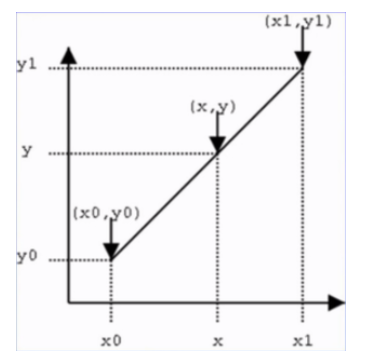通过斜率比，可以得到下面的等式。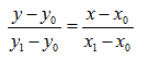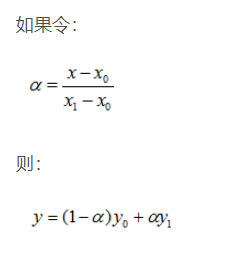双线性插值如图所示：所谓双线性插值，也就是连续使用三次一维线性插值，最终求得g(u0,v0)。 第一次：由g(u’,v’)和g(u’+1,v’)一维线性插值求g(u0,v’). 第二次：由g(u’,v’+1)和g(u’+1,v’+1)一维线性插值求g(u0,v’+1). 第三次：由g(u0,v’)和g(u0,v’+1)一维线性插值求g(u0,v0).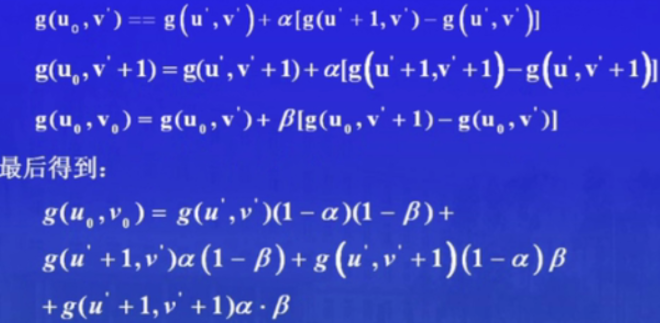如何用双线性插值放大或收缩图像
我们只需要做两件事：
计算新图像像素在原图像的对应位置通过位置，在原图像找到4个点来计算新图像对应位置的像素
f(x,y)表示输出图像，g(u,v)表示输入图像。图像放大或缩小的几何运算可定义为：

f

(

x

,

y

)

=

g

(

u

0

,

v

0

)

=

g

[

a

(

x

,

y

)

,

b

(

x

,

y

)

]

f(x,y)=g(u_0,v_0)=g[a(x,y),b(x,y)]

.如果令

u

0

=

a

(

x

,

y

)

=

x

c

,

v

0

=

b

(

x

,

y

)

=

y

d

u_0=a(x,y)=\frac{x}{c},v_0=b(x,y)=\frac{y}{d}

.我们知道

u

0

,

v

0

u_0,v_0

的坐标其实就是原图x轴方向放大c倍，y轴方向放大d被。
举个例子，将一副200×200的图像

g

(

u

,

v

)

g(u,v)

放大1.5倍，那么将得到300×300的新图像

f

(

x

,

y

)

f(x,y)

。产生新图像的过程，实际就是为300×300的像素赋值的过程。 假如为

f

(

150

,

150

)

f(150,150)

赋值：

f

(

150

,

150

)

=

g

(

150

/

1.5

,

150

/

1.5

)

=

g

(

100

,

100

)

;

f(150,150)=g(150/1.5,150/1.5)=g(100,100);

假如为f(100,100)赋值：

f

(

100

,

100

)

=

g

(

100

/

1.5

,

100

/

1.5

)

=

g

(

66.7

,

66.7

)

.

f(100,100)=g(100/1.5,100/1.5)=g(66.7,66.7).

对于

g

(

100

,

100

)

g(100,100)

,坐标值为整数，可直接赋值。而对于

g

(

66.7

,

66.7

)

g(66.7,66.7)

，坐标值为小数，也即

（

u

0

，

v

0

）

（u_0，v_0）

不一定在坐标点上，这种情况就需要用到前言中所提到的双线性插值。

f

(

100

,

100

)

=

g

(

66.7

,

66.7

)

=

(

0.3

∗

0.3

)

g

(

66

,

66

)

+

(

0.3

∗

0.7

)

g

(

66

,

67

)

+

(

0.3

∗

0.7

)

g

(

67

,

66

)

+

(

0.7

∗

0.7

)

g

(

67

,

67

)

f(100,100)=g(66.7,66.7)=(0.3*0.3)g(66,66)+(0.3*0.7)g(66,67)+(0.3*0.7)g(67,66)+(0.7*0.7)g(67,67)

python实现
# --*-- encoding: utf-8 --*--
'''
Date: 2018.09.13
Content: python3 实现双线性插值图像缩放算法
'''

import numpy as np
import cv2
import math

def bi_linear(src, dst, target_size):
th, tw = target_size, target_size
emptyImage = np.zeros(target_size, np.uint8)
for k in range(3):
for i in range(th):
for j in range(tw):
# 首先找到在原图中对应的点的(X, Y)坐标
corr_x = (i+0.5)/th*pic.shape-0.5
corr_y = (j+0.5)/tw*pic.shape-0.5
# if i*pic.shape%th==0 and j*pic.shape%tw==0:     # 对应的点正好是一个像素点，直接拷贝
#   emptyImage[i, j, k] = pic[int(corr_x), int(corr_y), k]
point1 = (math.floor(corr_x), math.floor(corr_y))   # 左上角的点
point2 = (point1, point1+1)
point3 = (point1+1, point1)
point4 = (point1+1, point1+1)

fr1 = (point2-corr_y)*pic[point1, point1, k] + (corr_y-point1)*pic[point2, point2, k]
fr2 = (point2-corr_y)*pic[point3, point3, k] + (corr_y-point1)*pic[point4, point4, k]
emptyImage[i, j, k] = (point3-corr_x)*fr1 + (corr_x-point1)*fr2

cv2.imwrite(dst, emptyImage)
# 用 CV2 resize函数得到的缩放图像
new_img = cv2.resize(pic, (200, 300))
cv2.imwrite('pic/1_cv_img.png', new_img)

def main():
src = 'pic/raw_1.jpg'
dst = 'pic/new_1.png'
target_size = (300, 200, 3)     # 变换后的图像大小

bi_linear(src, dst, target_size)

if __name__ == '__main__':
main()

展开全文算法 计算机视觉 opencv python
• 双线性插值算法的FPGA实现，Verilog代码，分享给大家一起学习！
• 自己写的双线性插值，处理图像马赛克，信噪比30db左右demosaic
• %读一幅图像 j=imrotate(i,30%图像旋转30度 k=imresize(i,2%图像放大两倍 t=imresize(i,2'bilinear%采用双线性插值法进行放大两倍 m=imresize(i,0.8%图像缩小到0.8倍 p=translate(strel(1, [25 25]%图像平移 img=...
• 能够实现对一幅图像的最近邻、双线性、双三次三种插值
• 实现一个图像缩放函数，可以对输入图像进行任意倍数的缩放...采用双线性插值进行重采样； X,Y方向的缩放倍数参函数参数的形式传入； 可以只考虑输入图像为3通道，8位深度的情况； 不能调用图像处理库的缩放函数来完成；
• 双线性插值图像放大 单通道图放大实现代码： #include<iostream> #include<opencv2/opencv.hpp> using namespace cv; //缩放图像函数 void enlargeImg(Mat src,Mat &dst,double size,int type) { ...
双线性插值图像放大
单通道图放大实现代码：
//op.h
#include<iostream>
#include<opencv2/opencv.hpp>

using namespace cv;

//缩放图像函数
void enlargeImg(Mat src,Mat &dst,double size,int type)
{
int dx = (int)((double)src.cols*(size-1));
int dy = (int)((double)src.rows*(size-1));
copyMakeBorder(src, dst, 0, dy, 0, dx, BORDER_CONSTANT);

if (type==0)//最近差值法
{
for(int i=0;i<dst.rows;i++)
{
for(int j=0;j<dst.cols;j++)
{
dst.at<uchar>(i,j)=src.at<uchar>(i/size,j/size);
}
}
}
else if(type==1)//双线性差值
{
double srcX,srcY;
double u,v;
for(int i=0;i<dst.rows;i++)
{
for(int j=0;j<dst.cols;j++)
{
srcX=(double)i/(double)size;
srcY=(double)j/(double)size;
u=srcX-floor(srcX);
v=srcY-floor(srcY);
int x=i/size,y=j/size;
dst.at<uchar>(i,j)=(int)((1-u)*(1-v)*(double)src.at<uchar>(x,y)+(1-u)*v*(double)src.at<uchar>(x,y+1)+u*(1-v)*(double)src.at<uchar>(x+1,y)+u*v*(double)src.at<uchar>(x+1,y+1));
}
}
}
}

//main.cpp
#include <iostream>
#include<opencv2/opencv.hpp>
#include"op.h"

#define path "dog.jpg"

using namespace cv;
using namespace std;

int main()
{

Mat dst;
imshow("源图像",src);
waitKey(0);
enlargeImg(src, dst, 3,0);//最近插值放大源图像3倍
imshow("双线性插值放大",dst);
waitKey(0);
enlargeImg(src, dst, 2.5,1);//双线性插值放大源图像2.5倍
imshow("双线性插值放大",dst);
waitKey(0);
return 0;
}


使用了大量的数据类型转换，代码效率不高，仅做到实现理论功能。
详细原理及代码优化参考：https://www.cnblogs.com/yssongest/p/5303151.html
展开全文opencv c++ 图像处理
• 目录双线性插值（Bilinear Interpolation）线性插值双线性插值深入理解双线性插值我的插值理解代码官方...首先双线性插值是用来放大图像的，但是我们都知道既然要放大图像，其实是对图像的像素进行扩充，而双线性插值


目录
双线性插值（Bilinear Interpolation）线性插值双线性插值
深入理解双线性插值我的插值理解代码官方插值办法源图像和目标图像几何中心的对齐将浮点运算转换成整数运算代码效果对比再次深入理解
引用

双线性插值（Bilinear Interpolation）
双线性插值是实现上采样的一种手段，关于它的知识其实非常非常简单，但是很多博客写的比较晦涩难懂，这里简单介绍一下双线性插值究竟做了什么。首先双线性插值是用来放大图像的，但是我们都知道既然要放大图像，其实是对图像的像素进行扩充，而双线性插值就是使用已有的像素点来计算其他像素点从而实现像素的扩充，也就是说双线性插值是在像素层面的计算。下面让我们先来看看什么是线性插值：
线性插值
先讲一下线性插值：已知数据 (x0, y0) 与 (x1, y1)，要计算 [x0, x1] 区间内某一位置 x 在直线上的y值（反过来也是一样，略）：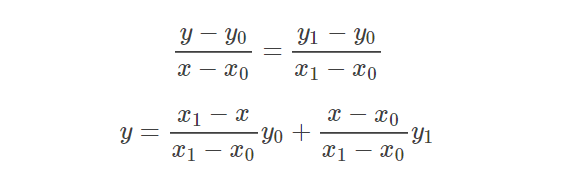上面比较好理解吧，仔细看就是用x和x0，x1的距离作为一个权重，用于y0和y1的加权。双线性插值本质上就是在两个方向上做线性插值。上面讲的实在是很清楚了，再来让我们看看双线性插值，其实没什么了不起的，就是从两个方向进行了插值。
双线性插值
这种方法特点是不需要进行学习，运行速度快，操作简单。只需要设置好固定的参数值即可，设置的参数就是中心值需要乘以的系数。双线性插值，这个名字咋一听很高大上的样纸，再在维基百科上一查（见文末，我去，一堆的公式吓死人），像俺这种半文盲，看到公式脑子就懵的类型，真心给跪。虽然看着好复杂，但仔细一看道理再简单不过了，所以还是自己梳理一下好。双线性插值，顾名思义就是两个方向的线性插值加起来（这解释过于简单粗暴，哈哈）。所以只要了解什么是线性插值，分别在x轴和y轴都做一遍，就是双线性插值了。如A点坐标(0,0),值为3，B点坐标(0,2)，值为5，那要对坐标为(0,1)的点C进行插值，就让C落在AB线上，值为4就可以了。但是如果C不在AB的线上肿么办捏，所以就有了双线性插值。如图，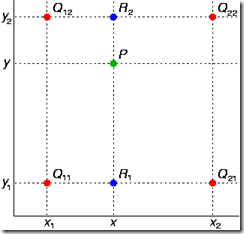已知Q12，Q22，Q11，Q21，但是要插值的点为P点，这就要用双线性插值了，首先在x轴方向上，对R1和R2两个点进行插值，这个很简单，然后根据R1和R2对P点进行插值，这就是所谓的双线性插值。方法：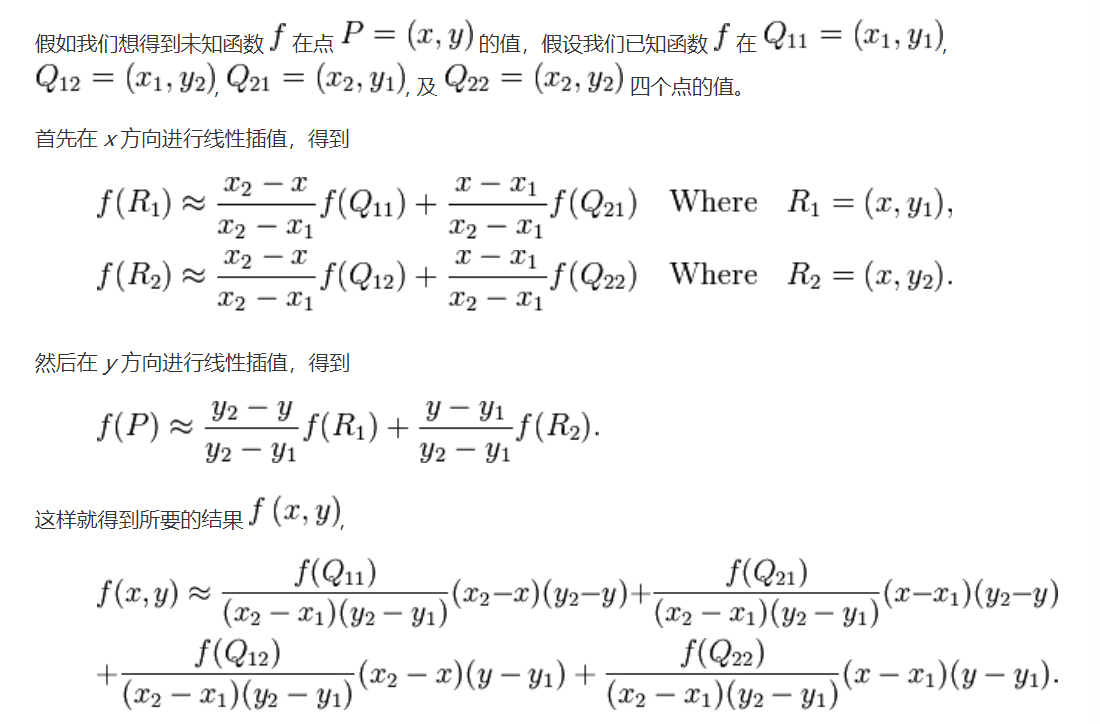咱们看一下完整公式推导：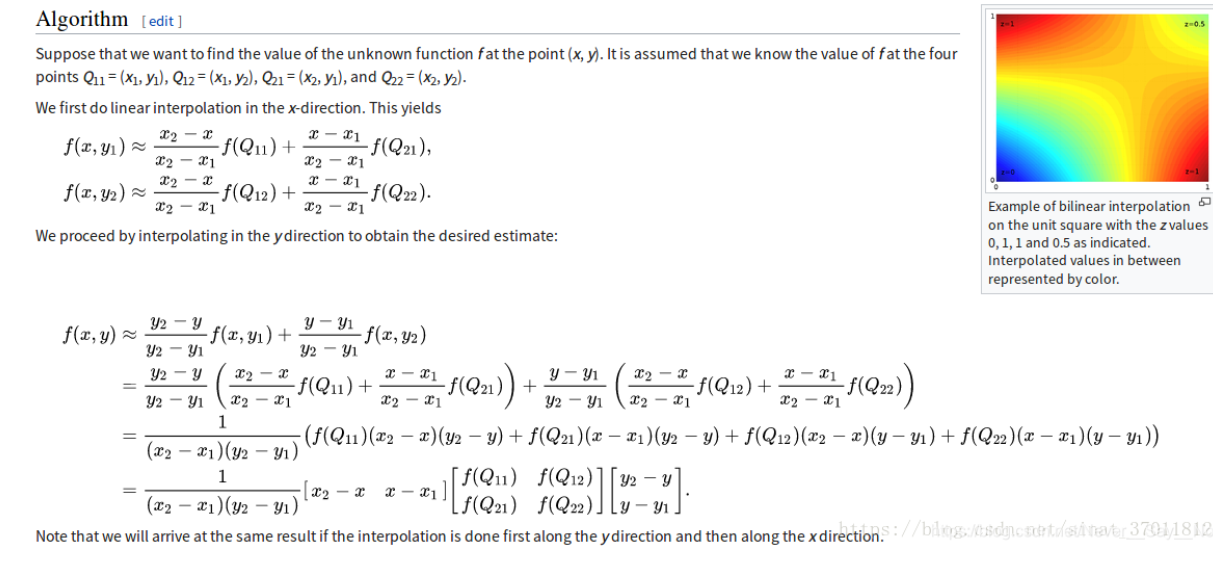下面重点来了：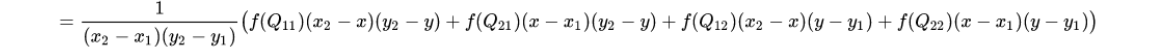由于咱们的像素间隔都是1，所以括号外面的分母(x2-x1)(y2-y1)=1，所以

上

式

=

f

(

Q

11

)

(

x

2

−

x

)

(

y

2

−

y

)

+

f

(

Q

21

)

(

x

−

x

1

)

(

y

2

−

y

)

+

f

(

Q

12

)

(

x

2

−

x

)

(

y

−

y

1

)

+

f

(

Q

22

)

(

x

−

x

1

)

(

y

−

y

1

)

上式=f(Q11)(x2-x)(y2-y)+f(Q21)(x-x1)(y2-y)+f(Q12)(x2-x)(y-y1)+f(Q22)(x-x1)(y-y1)

我们注意观察

(

x

2

−

x

)

(

y

2

−

y

)

(x2-x)(y2-y)

这个，其实

x

2

−

x

=

1

−

(

x

−

f

l

o

o

r

(

x

)

)

x2-x=1-(x-floor(x))

，floor是向下取整，细细品味一下你就会明白这个等式。同理，

x

−

x

1

=

x

−

f

l

o

o

r

(

x

)

x-x1=x-floor(x)

所以我们专门用

u

、

v

u、v

两个变量记录

x

−

f

l

o

o

r

(

x

)

，

y

−

f

l

o

o

r

(

y

)

x-floor(x)，y-floor(y)

，替换进去后，最终

上

式

=

f

(

Q

11

)

(

1

−

u

)

(

1

−

v

)

+

f

(

Q

21

)

u

(

1

−

v

)

+

f

(

Q

12

)

(

1

−

u

)

v

+

f

(

Q

22

)

u

v

上式=f(Q11)(1-u)(1-v)+f(Q21)u(1-v)+f(Q12)(1-u)v+f(Q22)uv

深入理解双线性插值
我的插值理解
通过上面的例子，其实我们能够有所感觉，所谓的插值，不过是通过周围已知的像素值来计算一些原本没有给出的像素值；所谓的线性，是指我们通过线性的方式来用已知像素值计算未知的像素值。这样的话像素值会增多，也就实现了图像的放大。当然我们也可以进行图像的缩小，一样的道理，还是进行插值，只不过需要的像素点少了而已。这样看来的话，给我们一个像素矩阵，我们应当也是会根据要求来进行插值的。现在我们来看一个图像放大的例子：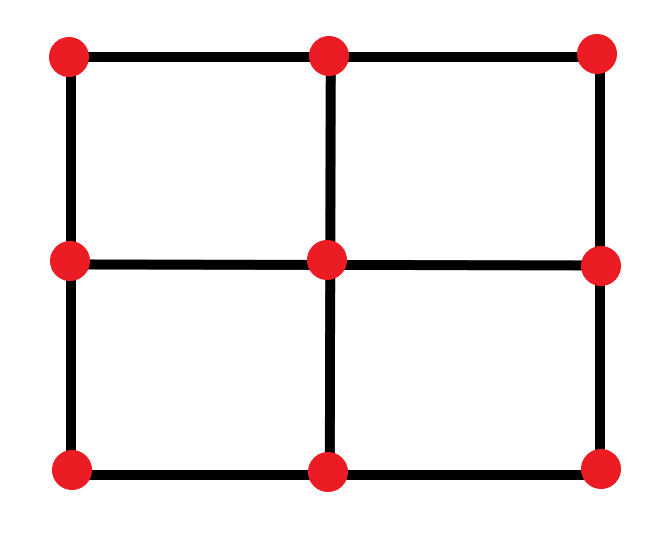上图是原来的图像，是3*3的矩阵，而我们的任务是放大到4*4，我们首先需要建立一个坐标系，左上角为（0,0）。通过图像我们可以看到，我们要放大图像的话，其实边界都是之前图像的边界，我们只不过是将原图的边划分成更小的粒度而已，比如上图原来元素之间间隔1，共有3个元素，但我们要想在原来的长度下构成4个元素，那每个元素间隔就是2/3，新矩阵间隔计算公式为**(每边原来的元素数-1)/(目标每边的元素数-1)**，所以我们就可以画出下面的新矩阵：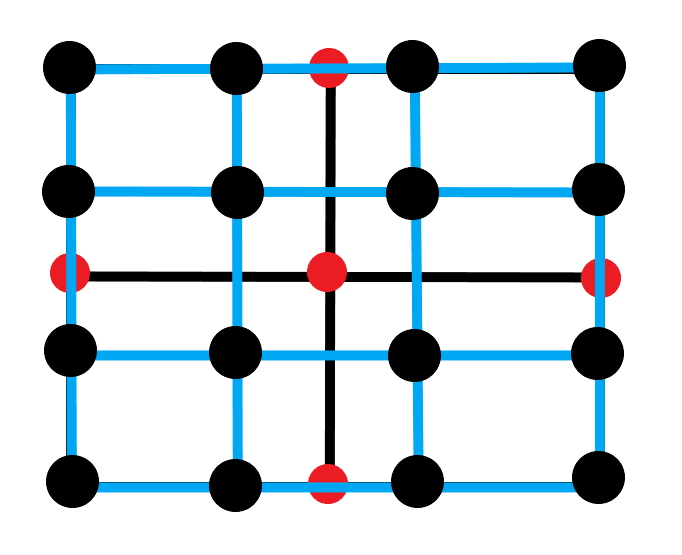黑点的间隔就是2/3，然后利用双线性插值的公式来计算上图每个黑点的像素值就好了。
代码
from PIL import Image
import matplotlib.pyplot as plt
import numpy as np
import math
def BiLinear_interpolation(img,dstH,dstW):
scrH,scrW,_=img.shape
print(img.shape)
print(img.shape)
retimg=np.zeros((dstH,dstW,3),dtype=np.uint8)
for i in range(dstH):
for j in range(dstW):
scrx=i*(scrH-1)/(dstH-1)
scry=j*(scrW-1)/(dstW-1)
x=math.floor(scrx)
y=math.floor(scry)
u=scrx-x
v=scry-y
retimg[i,j]=(1-u)*(1-v)*img[x,y]+u*(1-v)*img[x+1,y]+(1-u)*v*img[x,y+1]+u*v*img[x+1,y+1]
return retimg
im_path='testPict.png'
image=np.array(Image.open(im_path))
image2=BiLinear_interpolation(image,image.shape*10,image.shape*10)
image2=Image.fromarray(image2.astype('uint8')).convert('RGB')
image2.save('outmy_x10.png')

官方插值办法
emmm，怎么说呢，如果你读懂了我的理解之后，你应该知道，我的方法其实是相当于在原图的内部提取出更多的点，从而扩大图像。下面我用同样的例子来展示官方差值的作法：下面是将3*3扩大成4*4的图像：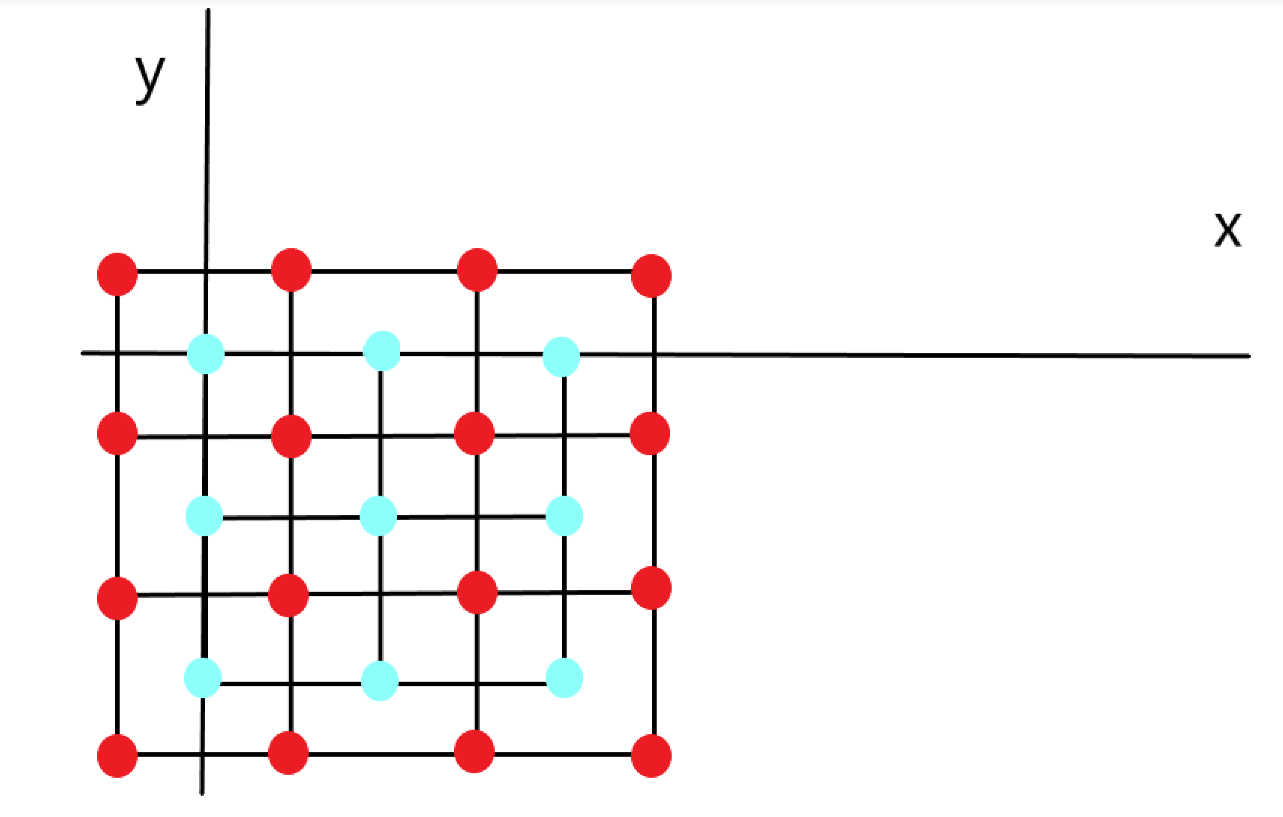有人可能会说，那边际的红点怎么推测呀，周围的蓝色的点不够4个啊，请看下图：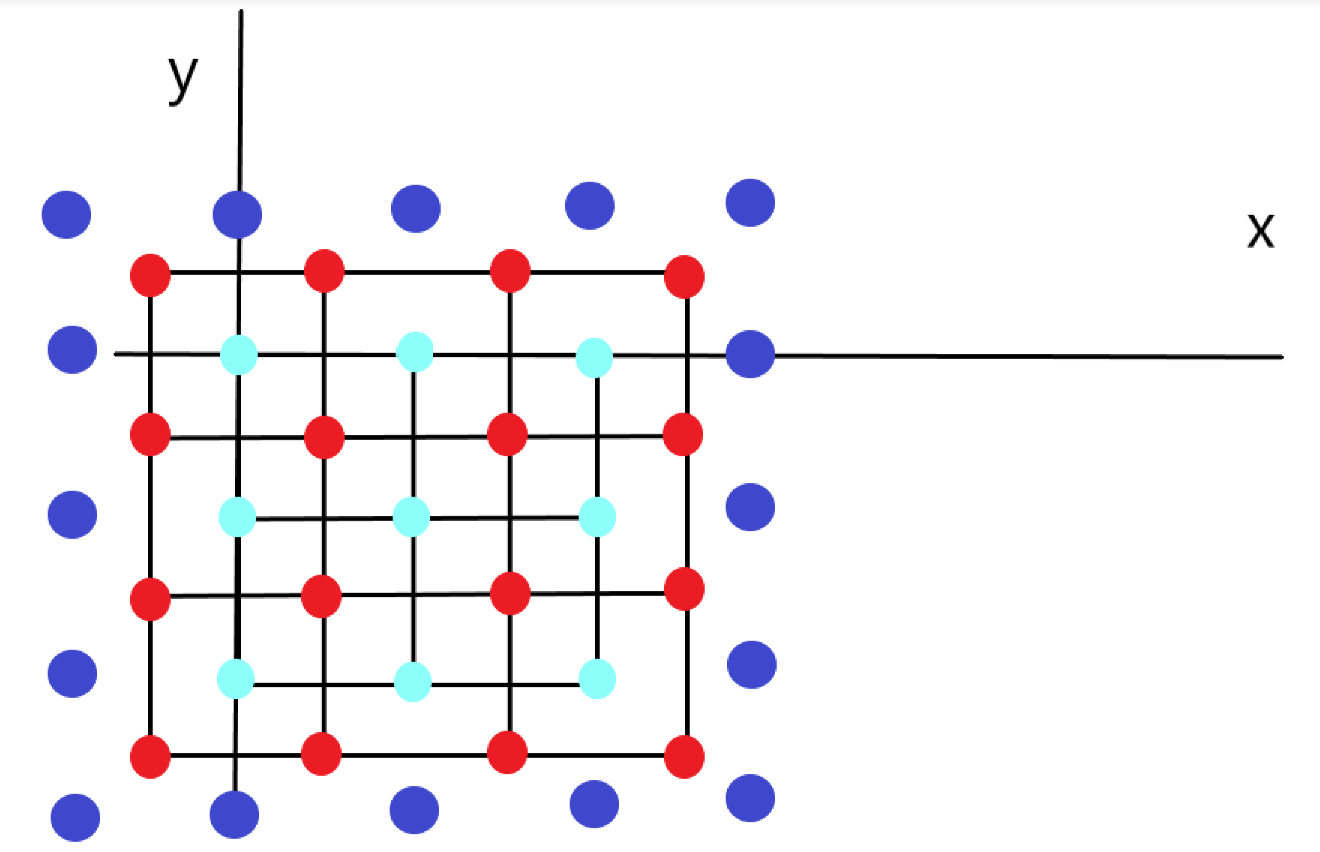猜一下深蓝色的像素点是什么，没错，就是padding的像素点，像素值为0，也就是说只需要padding就好了。那么如何根据原图计算目标图的像素点分别对应原图的坐标呢？ srcX=dstX* (srcWidth/dstWidth), srcY = dstY* (srcHeight/dstHeight) 其中(dstX，dsY）是目标图像的像素点的逻辑下标，左上角的点规定为（0，0），srcWidth是原图像的每边像素点的个数，dstWidth是目标图像的每条边像素点的个数，srcX是目标图像像素点（dstX，dstY）对应在原图像的坐标系下的实际坐标，可能不是整数，那就需要插值了。 经过几何中心对齐后的优化后的公式为： SrcX=(dstX+0.5)* (srcWidth/dstWidth) -0.5 SrcY=(dstY+0.5) * (srcHeight/dstHeight)-0.5
源图像和目标图像几何中心的对齐
这是优化措施之一，针对官方的双线性插值方法，在我的方法中双线性插值一开始就是几何中心对齐的。按照网上一些博客上写的，源图像和目标图像的原点（0，0）均选择左上角，然后根据插值公式计算目标图像每点像素，假设你需要将一幅5x5的图像缩小成3x3，那么源图像和目标图像各个像素之间的对应关系如下：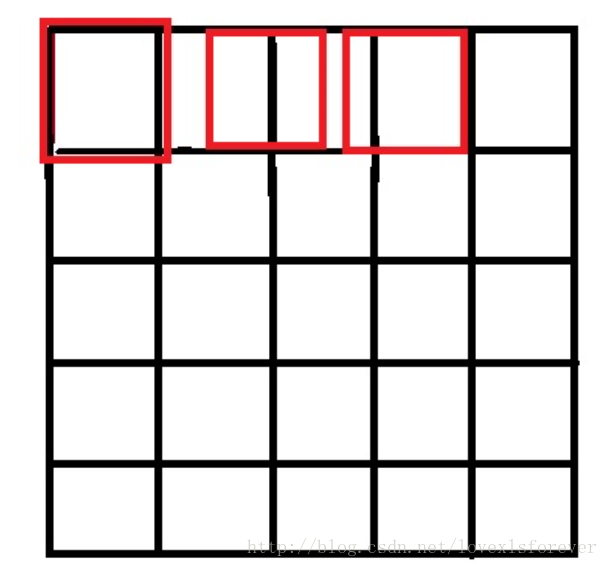只画了一行，用做示意，从图中可以很明显的看到，如果选择右上角为原点（0，0），那么最右边和最下边的像素实际上并没有参与计算，而且目标图像的每个像素点计算出的灰度值也相对于源图像偏左偏上。 那么，让坐标加1或者选择右下角为原点怎么样呢？很不幸，还是一样的效果，不过这次得到的图像将偏右偏下。 最好的方法就是，两个图像的几何中心重合，并且目标图像的每个像素之间都是等间隔的，并且都和两边有一定的边距，这也是matlab和openCV的做法。如下图：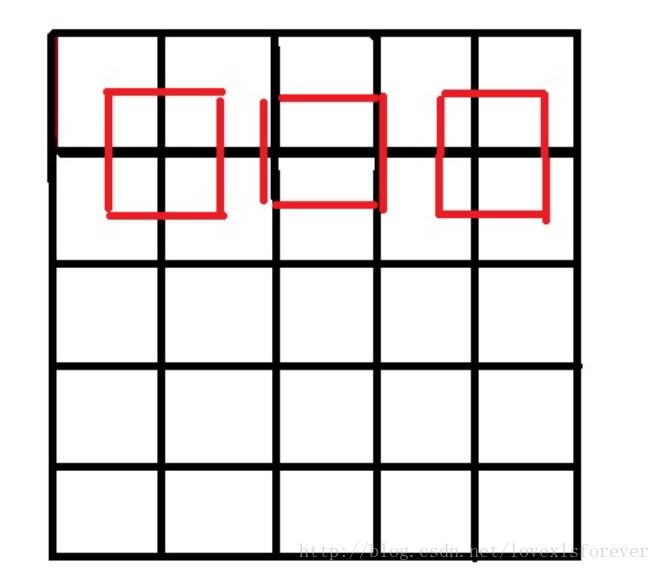分别使用上述提到的公式和几何中心对齐之后的公式进行处理后，就会分别得到上述对应的两幅图，显然使用几何中心对齐的公式更为合理。看个例子：假设源图像是33，中心点坐标（1，1）目标图像是99，中心点坐标（4，4），我们在进行插值映射的时候，尽可能希望均匀的用到源图像的像素信息，最直观的就是（4,4）映射到（1,1）现在直接计算srcX=4*3/9=1.3333！=1，也就是我们在插值的时候所利用的像素集中在图像的右下方，而不是均匀分布整个图像。现在考虑中心点对齐，srcX=(4+0.5)*3/9-0.5=1，刚好满足我们的要求。
将浮点运算转换成整数运算
这也是优化方法之一。参考图像处理界双线性插值算法的优化，直接进行计算的话，由于计算的srcX和srcY 都是浮点数，后续会进行大量的乘法，而图像数据量又大，速度不会理想，解决思路是： 　　浮点运算→→整数运算→→”<<左右移按位运算”。 放大的主要对象是u，v这些浮点数，OpenCV选择的放大倍数是2048“如何取这个合适的放大倍数呢，要从三个方面考虑， 　　第一：精度问题，如果这个数取得过小，那么经过计算后可能会导致结果出现较大的误差。 　　第二，这个数不能太大，太大会导致计算过程超过长整形所能表达的范围。 　　第三：速度考虑。假如放大倍数取为12，那么算式在最后的结果中应该需要除以1212=144，但是如果取为16，则最后的除数为1616=256，这个数字好，我们可以用右移来实现，而右移要比普通的整除快多了。”我们利用左移11位操作就可以达到放大目的。
代码
from PIL import Image
import matplotlib.pyplot as plt
import numpy as np
import math
def BiLinear_interpolation(img,dstH,dstW):
scrH,scrW,_=img.shape
print(img.shape)
print(img.shape)
retimg=np.zeros((dstH,dstW,3),dtype=np.uint8)
for i in range(dstH):
for j in range(dstW):
scrx=(i+0.5)*(scrH/dstH)-0.5
scry=(j+0.5)*(scrW/dstW)-0.5
x=math.floor(scrx)
y=math.floor(scry)
u=scrx-x
v=scry-y
retimg[i,j]=(1-u)*(1-v)*img[x,y]+u*(1-v)*img[x+1,y]+(1-u)*v*img[x,y+1]+u*v*img[x+1,y+1]
return retimg
im_path='testPict.png'
image=np.array(Image.open(im_path))
image2=BiLinear_interpolation(image,image.shape*10,image.shape*10)
image2=Image.fromarray(image2.astype('uint8')).convert('RGB')
image2.save('out0.5_x10.png')

效果对比
我分别运行了下，其实几乎分辨不出来任何区别……不过用我的方法得到的图像占空间比较大。
再次深入理解
在图像的仿射变换中，很多地方需要用到插值运算，常见的插值运算包括最邻近插值，双线性插值，双三次插值，兰索思插值等方法，OpenCV提供了很多方法，其中，双线性插值由于折中的插值效果和运算速度，运用比较广泛。 　　越是简单的模型越适合用来举例子，我们就举个简单的图像：3*3 的256级灰度图。假如图像的象素矩阵如下图所示（这个原始图把它叫做源图，Source）： 234 38 22 67 44 12 89 65 63 　　这 个矩阵中，元素坐标(x,y)是这样确定的，x从左到右，从0开始，y从上到下，也是从零开始，这是图象处理中最常用的坐标系。 　　如果想把这副图放大为 4*4大小的图像，那么该怎么做呢？那么第一步肯定想到的是先把4*4的矩阵先画出来再说，好了矩阵画出来了，如下所示，当然，矩阵的每个像素都是未知数，等待着我们去填充（这个将要被填充的图的叫做目标图,Destination）： 　　? ? ? ? 　　? ? ? ? 　　? ? ? ? 　　? ? ? ? 　　然后要往这个空的矩阵里面填值了，要填的值从哪里来来呢？是从源图中来，好，先填写目标图最左上角的象素，坐标为（0，0），那么该坐标对应源图中的坐标可以由如下公式得出srcX=dstX* (srcWidth/dstWidth) , srcY = dstY * (srcHeight/dstHeight) 　　好了，套用公式，就可以找到对应的原图的坐标了(0*(3/4),0*(3/4))=>(0*0.75,0*0.75)=>(0,0)，找到了源图的对应坐标,就可以把源图中坐标为(0,0)处的234象素值填进去目标图的(0,0)这个位置了。 　　接下来,如法炮制,寻找目标图中坐标为(1,0)的象素对应源图中的坐标,套用公式: (1*0.75,0*0.75)=>(0.75,0) 结果发现,得到的坐标里面竟然有小数,这可怎么办?计算机里的图像可是数字图像,象素就是最小单位了,象素的坐标都是整数,从来没有小数坐标。这时候采用的一种策略就是采用四舍五入的方法（也可以采用直接舍掉小数位的方法），把非整数坐标转换成整数，好，那么按照四舍五入的方法就得到坐标（1，0），完整的运算过程就是这样的：(1*0.75,0*0.75)=>(0.75,0)=>(1,0) 那么就可以再填一个象素到目标矩阵中了，同样是把源图中坐标为(1,0)处的像素值38填入目标图中的坐标。 　　依次填完每个象素，一幅放大后的图像就诞生了，像素矩阵如下所示： 　　234 38 22 22 　　67 44 12 12 　　89 65 63 63 　　89 65 63 63 　　这种放大图像的方法叫做最临近插值算法，这是一种最基本、最简单的图像缩放算法，效果也是最不好的，放大后的图像有很严重的马赛克，缩小后的图像有很严重的失真；效果不好的根源就是其简单的最临近插值方法引入了严重的图像失真，比如，当由目标图的坐标反推得到的源图的的坐标是一个浮点数的时候，采用了四舍五入的方法，直接采用了和这个浮点数最接近的象素的值，这种方法是很不科学的，当推得坐标值为 0.75的时候，不应该就简单的取为1，既然是0.75，比1要小0.25 ，比0要大0.75 ,那么目标象素值其实应该根据这个源图中虚拟的点四周的四个真实的点来按照一定的规律计算出来的，这样才能达到更好的缩放效果。 　　双线型内插值算法就是一种比较好的图像缩放算法，它充分的利用了源图中虚拟点四周的四个真实存在的像素值来共同决定目标图中的一个像素值，因此缩放效果比简单的最邻近插值要好很多。 双线性内插值算法描述如下: 　　对于一个目的像素，设置坐标通过反向变换得到的浮点坐标为(i+u,j+v) (其中i、j均为浮点坐标的整数部分，u、v为浮点坐标的小数部分，是取值[0,1)区间的浮点数)，则这个像素得值 f(i+u,j+v) 可由原图像中坐标为 (i,j)、(i+1,j)、(i,j+1)、(i+1,j+1)所对应的周围四个像素的值决定，即：f(i+u,j+v) = (1-u)(1-v)f(i,j) + (1-u)vf(i,j+1) + u(1-v)f(i+1,j) + uvf(i+1,j+1) 其中f(i,j)表示源图像(i,j)处的的像素值，以此类推。 　　比如，象刚才的例子，现在假如目标图的象素坐标为（1，1），那么反推得到的对应于源图的坐标是（0.75 , 0.75）, 这其实只是一个概念上的虚拟象素,实际在源图中并不存在这样一个象素,那么目标图的象素（1，1）的取值不能够由这个虚拟象素来决定，而只能由源图的这四个象素共同决定：（0，0）（0，1）（1，0）（1，1），而由于（0.75,0.75）离（1，1）要更近一些，那么（1,1）所起的决定作用更大一些，这从公式1中的系数uv=0.75×0.75就可以体现出来，而（0.75,0.75）离（0，0）最远，所以（0，0）所起的决定作用就要小一些，公式中系数为(1-u)(1-v)=0.25×0.25也体现出了这一特点。
引用
https://blog.csdn.net/qq_37577735/article/details/80041586https://blog.csdn.net/never__say__no/article/details/109081233https://www.cnblogs.com/wojianxin/p/12516029.html
展开全文算法 计算机视觉
• 双线性插值放大缩小图片，即可运行，mfc程序，vs2008开发环境
•Matlab 图像缩放
• 1. 线性插值 线性插值是数学、计算机图形学等领域广泛使用的一种简单插值方法。 假设我们已知坐标 ((x0,y0) 与 (x1,y1)，要得到 [x0,x1] 区间内某一位置x在直线上的值。...2. 双线性插值(Bilinear...
• 纯c语言实现bmp图像的双线性插值放大，最近邻插值放大
• 最近学数值分析的时候，手写了一下图像双线性插值算法，主要思路是对插值后的每个像素点进行计算，然后这些点构成了插值后的图像。逐个像素点计算速度比较慢，下面公式推导给出了矩阵形式，若采用矩阵运算应该比较快...算法 python
• 图像的放大本质上就是增加像素点，目前常用的传统方法是内插法，代表算法有最临近点插值算法、双线性插值算法和双三次插值法，这些算法都是基于相邻像素点的像素值计算所要增加的像素点的像素值，因而在放大时会有...
• 彩色图像插值放大 最近邻插值 双线性插值 双立方插值
• C# 双线性插值放大图像 C# 双线性插值放大图像 C# 双线性插值放大图像
• matlab开发-图像处理使用双线性插值缩放动画。本文采用双线性插值法对图像进行正整数因子缩放。
• 要对其进行改进，对图像的缩小则可以用“局部均值法”，对于图像的放大则可以用“双线性插值法”。 效果如下： 2048*1536缩小为100*80时的效果 100*80放大到600*400的效果 局部均值法缩小图像 （1）计算...
• 双线性插值法原理： ① 何为线性插值插值就是在两个数之间插入一个数，线性插值原理图如下： 在位置 x 进行线性插值，插入的值为f(x) ↑ ② 各种插值法： 插值法的第一步都是相同的，计算目标图...数字图像处理 opencv
• 利用C++实现了最近邻插值以及双线性插值的图像插值算法，将两种算法并成一个API，可以加深对于这两个插值算法的理解
• 一、实验目的  1、熟悉并掌握MATLAB工具的使用...2、对图像执行放大、缩小及旋转操作，分别采用最近邻插值双线性插值及双三次插值方法实现，要求根据算法自己编写代码实现，并分析三种方法的优缺点。 （二）、相关知matlab 图像处理
• 该文档里包含verilog语言编写的双线性插值实现图像缩放的算法...